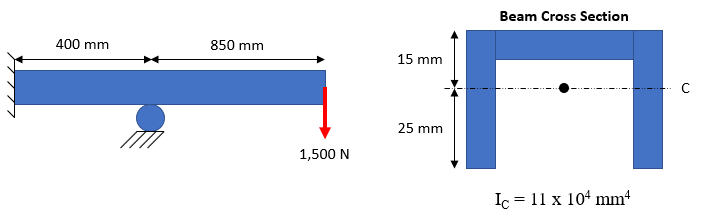## Bending Tensile Stress

In the below figure, a beam is loaded by hanging a 1,500 N downward force. What is the tensile stress due to bending?Hint
Because there is a fixed roller towards the middle section of the beam, the max bending moment occurs on the beam's right portion:
$$Max\:Bending\:Moment=force\times distance$$$Hint 2 The normal stress in a beam due to bending: $$\sigma_x=\frac{My}{I}$$$
where $$M$$ is the moment at the section, $$I$$ is the moment of inertia of the cross section, and $$y$$ is the distance from the neutral axis to the fiber location above or below the neutral axis.
Because there is a fixed roller towards the middle section of the beam, the max bending moment occurs on the beam's right portion:
$$Max\:Bending\:Moment=force\times distance$$$$$=1500N\times 850mm=1.275\cdot 10^6\:N\cdot mm$$$
The normal stress in a beam due to bending:
$$\sigma_x=\frac{My}{I}$$$where $$M$$ is the moment at the section, $$I$$ is the moment of inertia of the cross section, and $$y$$ is the distance from the neutral axis to the fiber location above or below the neutral axis. $$\sigma_{tension}=\frac{(1.275\cdot 10^6N\cdot mm)(15mm)}{11\cdot 10^4mm^4}=174\:MPa$$$
174 MPa